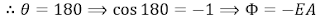# The Electric Flux & Gauss's Law

### The Electric Flux:

The electric flux is the total number of electric field's lines "E" penetrating a surface of area "A"

### The area vector:

The area vector has a magnitude and a direction that is always perpendicular to the area towards the outside.

### The Cases of the angle:

 If the electric field is perpendicular to the area vectorIf the electric field is parallel to the area vector (Same direction)If the electric field is parallel to the area vector (opposite direction)If the electric field is no perpendicular nor parallel to the area vector### Gauss's law:

It describes the relationship of electric flux (through a closed surface) to the positive charge (within that closed surface).

### Notes:-

1- The previous law means that the electric flux through any closed surface around a positive charge is not affected by the shape of that surface.

2- The direction of the electric field is perpendicular to the area, and parallel to the area vector (in the same direction).

### Conditions for applying Gauss's law:-

1- The charge is evenly distributed

2- The surface is closed (ball, cylinder ...)

### Calculating the Electric Field through a Gaussian surface:-

Case 1: if the Gaussian surface is a ball: - The electric field at any point is

Case 2: if the Gaussian surface is a cylinder: - The electric field at any point is